• 在回归分析中，只包括一个自变量和一个因变量，且二者的关系可用一条直线近似表示，这种回归分析称为一元线性回归分析。如果回归分析中包括两个或两个以上的自变量，且因变量和自变量之间是线性关系，则称为多元线性...
线性回归（一）
线性回归是分析因变量与自变量呈现线性关系的一种方法，来确定一个因变量如何依赖一个或多个自变量的变化而变化，运用十分广泛。
在回归分析中，只包括一个自变量和一个因变量，且二者的关系可用一条直线近似表示，这种回归分析称为一元线性回归分析。如果回归分析中包括两个或两个以上的自变量，且因变量和自变量之间是线性关系，则称为多元线性回归分析。
线性回归常用参数：
regression.intercept_  ：    截距，  默认为True，可选False
regression.coef_  ：    斜率，回归系数，x对y的具体影响
regression.predict：    预测
normalize：   （标准化） 默认为True，可选False
copy_X：   （复制X数据）默认为True，可选False。如果选False会覆盖原数
n_jobs：   （计算性能）默认为1，可选int，工作使用的数量计算。
一元线性回归
from sklearn import linear_model #导入线性模型模块
regression = linear_model.LinearRegression()  #创建线性回归模型
x = [,]    #创建x坐标
y = [6,22]        #创建y坐标
regression.fit(x,y)  #拟合


LinearRegression(copy_X=True, fit_intercept=True, n_jobs=1, normalize=False)

regression.intercept_   #截距，以下划线结束

-0.8571428571428559

regression.coef_    #斜率，回归系数，x对y的具体影响

array([2.28571429])

regression.predict([])  #对未知点进行预测

array([12.85714286])

regression.score([,,,],[12.8,14.6,17.8,19.5])   #返回可决系数R2

0.9825215658041578

#练习
regression1 = linear_model.LinearRegression()  #创建线性回归模型
x1 = [,,,]    #创建x坐标
y1 = [6,22,24,29]        #创建y坐标
regression1.fit(x1,y1)  #拟合
regression1.intercept_


0.8910256410256423

regression1.coef_

array([1.93589744])

regression1.predict([,,])

array([12.50641026, 14.44230769, 16.37820513])

regression1.score([,,,],[12.8,14.6,17.8,19.5])

0.8716272717012157

# 案例：假如研究学生学习时长(分钟)与考试成绩（分）的关系，考试成绩为因变量，学习时长为自变量，首先导入回归模块
import pandas as pd #导入pandas库
import numpy as np #导入numpy库
#from sklearn import linear_model #导入线性模型模块
#regression = linear_model.LinearRegression()  #创建线性回归模型，导入线性模块也可采用下列方式一次性导入
from sklearn.linear_model import LinearRegression #导入机器学习库中的线性回归模块
data=pd.DataFrame({'times':[150,200,250,300,350,400,600],
'scores':[64,68,75,79,85,89,93]})
#创建一组7行2列的数据，times为学习时长，scores为对应成绩


data_train=np.array(data['times']).reshape(data['times'].shape,1)#这里是将数据转化为一个1维矩阵
data_test=data['scores']
#创建线性回归模型，拟合学习时长与学习成绩的关系，并预测成绩
reg1=LinearRegression() #创建线性回归模型，参数默认
reg1.fit(data_train,data_test)#拟合数据
a=reg1.predict(268.5)  #预测学习时长为268.5分钟的学习成绩
print(a)#查看预测结果
print(reg1.score(data_train,data_test))#查看拟合准确率情况,这里的检验是 R^2 ，趋近于1模型拟合越好


[75.43295213]
0.8788360721074254

#预测的结果：学习时长为268.5分钟，则对应的学习成绩为75.43分， R^2 =0.8788
#我们来画个图看一下数据最后是什么样的
import matplotlib.pyplot as plt
plt.scatter(data['times'],data['scores']) #画散点图
plt.plot(data['times'],reg1.predict(np.array(data['times']).reshape(data['times'].shape,1)),color='red') #画拟合线型图


[<matplotlib.lines.Line2D at 0x21c131f8128>]展开全文• UA MATH571A 一元线性回归III 一元线性回归的ANOVAANOVA TableF检验回归系数的F检验F检验与t检验等价广义线性检验方法R2R^2R2数值例子：女性肌肉量与年龄的关系 ANOVA Table ANOVA（Analysis of Variance）是分析...
UA MATH571A 一元线性回归III 方差分析与相关性分析ANOVA TableF检验回归系数的F检验F检验与t检验等价广义线性检验方法$R^2$数值例子：女性肌肉量与年龄的关系相关性系数PPMCCPPMCC的区间估计Spearman秩相关系数数值例子：学历与犯罪率
ANOVA Table
ANOVA（Analysis of Variance）是分析方差构成的常用方法。在前两篇中，我们定义过
$SST = \sum_{i=1}^N (Y_i-\bar{Y})^2$
SST表示被解释变量Y的样本总离差平方和（或称总平方和），代表样本数据整体的信息含量，其自由度为$df_T=N-1$。我们也定义过
$SSE = \sum_{i=1}^{N} e_i^2 = \sum_{i=1}^{N} (Y_i - \hat{Y}_i)^2$
SSE是回归的残差平方和，代表无法被变量X解释的那部分信息量，自由度为$df_E=N-2$。
$SST-SSE=\sum_{i=1}^N [(Y_i-\bar{Y})^2-(Y_i - \hat{Y}_i)^2] \\ =\sum_{i=1}^N [\bar{Y}^2+\hat{Y_i}^2-2Y_i(\hat{Y}_i-\bar{Y})] \\ = \sum_{i=1}^N [\bar{Y}^2+\hat{Y_i}^2-2(Y_i-\bar{Y})(\hat{Y}_i-\bar{Y})] \\ =\sum_{i=1}^N (\hat{Y}_i - \bar{Y})^2 \triangleq SSR$
SSR是回归平方和，代表回归模型可以解释的那部分信息含量，自由度为$df_R=1$。对于回归而言，只有两个回归系数贡献两个自由度，但存在约束$\sum_{i=1}^N (\hat{Y}_i - \bar{Y})=0$，所以减去一个自由度，只剩下一个自由度。将三个平方和做自由度修正，定义
$MST = \frac{SST}{df_T}, \ \ MSR = \frac{SSR}{df_R}, \ \ MSE = \frac{SSE}{df_E}$
根据上述定义，可以写出下列方差分析表（ANOVA Table）

来源
SS
df
MS

回归
$SSR=\sum_{i=1}^N (\hat{Y}_i - \bar{Y})^2$
1
$MSR = \frac{SSR}{df_R}$

残差
$SSE=\sum_{i=1}^N (Y_i - \hat{Y}_i )^2$
N-2
$MSE = \frac{SSE}{df_E}$

总平方和
$SST=\sum_{i=1}^N (Y_i - \bar{Y})^2$
N-1
$MST = \frac{SST}{df_T}$

F检验
回归系数的F检验
之前有说过MSE是方差的无偏估计，也就是$E(MSE)=\sigma^2$。现在计算一下MSR的期望。
$SSR = \sum_{i=1}^N (\hat{Y}_i - \bar{Y})^2 = \sum_{i=1}^N [\hat{\beta}_0 +\hat{\beta}_1X_i- (\hat{\beta}_0+\hat{\beta}_1\bar{X})]^2 =\hat{\beta}_1^2\sum_{i=1}^N (X_i - \bar{X})^2 \\ E(\hat{\beta}_1^2)=Var(\hat{\beta}_1)+[E(\hat{\beta}_1)]^2=\frac{\sigma^2}{\sum_{i=1}^N (X_i - \bar{X})^2} + \beta_1^2 \\ E(MSR)=E(SSR)=\sigma^2 + \beta_1^2 \sum_{i=1}^N (X_i - \bar{X})^2$
显然当$\beta_1$等于0时，MSR也是方差的无偏估计，当$\beta_1$不等于0时，MSR不是方差的无偏估计。考虑对系数的双边检验：
$H_0: \beta_1 = 0 \\ H_a: \beta_1 \ne 0$
定义统计量
$F^* = \frac{MSR}{MSE}$
$SSR/\sigma^2$是标准正态随机变量的平方，由于自由度为1，因此服从$\chi^2(1)$分布，所以根据F分布的定义，在原假设下，$F^* \sim (1,N-2)$。假设检验水平为$\alpha$，若$F^*\le F(1-\alpha;1,N-2)$，接受原假设，若$F^*>F(1-\alpha;1,N-2)$，拒绝原假设。
F检验与t检验等价
F检验与双边t检验等价，
$F^* = \frac{MSR}{MSE}=\frac{SSR/1}{MSE}=\frac{\hat{\beta}_1^2\sum_{i=1}^N (X_i - \bar{X})^2}{MSE}=\frac{\hat{\beta}_1^2}{s^2\{\hat{\beta}_1\}}=(t^*)^2$
但由于F分布是单尾分布，因此与t检验不同，F检验只能做双边检验。
广义线性检验方法
完整的一元线性回归模型为FM（Full Model）：
$Y_i = \beta_0 + \beta_1 X_i + \epsilon_i$
其残差平方和为
$SSE(FM)=\sum_{i=1}^N (Y_i - \hat{Y}_i )^2 = \sum_{i=1}^N [Y_i -( \hat{\beta}_0 + \hat{\beta}_1\hat{X}_i )]^2 =SSE$
在原假设下，$\beta_1$等于0，完整的一元回归模型可以被简化为RM（Reduced Model）：
$Y_i = \beta_0 + \epsilon_i$
残差平方和为
$SSE(RM)=\sum_{i=1}^N (Y_i - \hat{Y}_i )^2 = \sum_{i=1}^N (Y_i - \hat{\beta}_0 )^2 = \sum_{i=1}^N (Y_i - \bar{Y})^2 =SST$
在这些设定下，可以将F检验推广。定义
$F^* = \frac{SSE(RM)-SSE(FM)}{df_{RM}-df_{FM}}/\frac{SSE(FM)}{df_{FM}} \sim F(df_{RM}-df_{FM},df_{FM})$
原假设为应该使用RM，备择假设为应该使用FM。
$R^2$
$R^2$表示能够用回归模型解释的那部分信息占总信息的比值，
$R^2 = \frac{SSR}{SST}=1-\frac{SSE}{SST}$
$R^2$又叫可决系数，$R^2$越大代表回归模型越能解释被解释变量Y的变化情况，回归模型质量就越高。
数值例子：女性肌肉量与年龄的关系
我们最后再用这个例子来介绍一下做ANOVA的F检验的方法，关于这个例子已经完成的分析可以看前两篇博文。对线性模型lm()的输出结果使用anova()函数可以得到ANOVA Table，
> anova(Ex1.lm)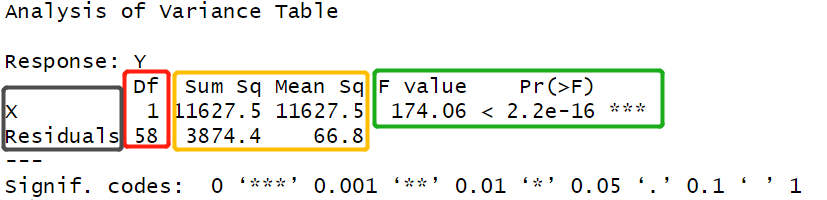灰框中是ANOVA Table中的方差来源栏，红框中是自由度，黄框中是SS和MS。绿框中是F统计量和F检验的p值，根据这两个值可以判断回归系数$\beta_1$是显著异于0的，说明回归有效，这与t检验的结果一致。在回归结果的汇总中，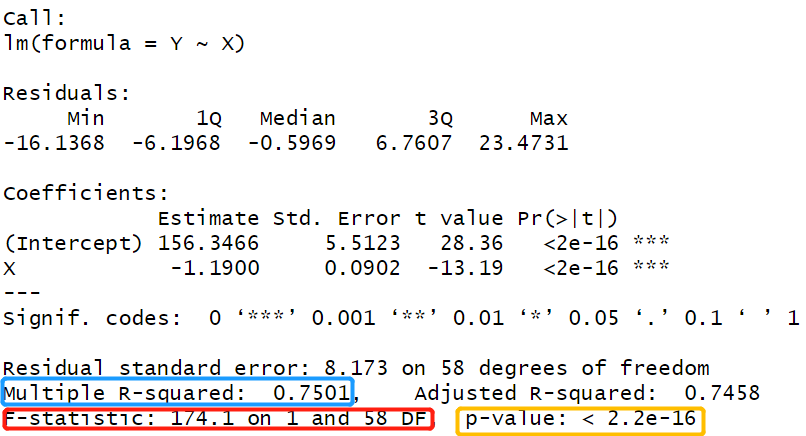红框内的是F统计量及其对应的自由度，黄框内是F检验的p值，这与ANOVA Table中的结果一致。简单计算可以发现$\beta_1$的t统计量的平方等于F统计量，但t统计量可以有正负，而F统计量总是为正的，这是因为t分布是双尾分布，而F分布只有单尾。因此做单边检验时只能用t检验。蓝框内的值是$R^2$，这个值说明年龄可以解释女性肌肉量75%的变化。但要注意的是解释不代表因果，只是一个统计相关性。这个结果只能说明女性肌肉量的下降从统计上讲有75%与年龄增长有关，但不能证明女性肌肉量的下降有75%是年龄增长造成的。
相关性系数
在回归模型中，我们认为变量X的改变会引起变量Y的改变（称这种关系是统计上的因果关系），变量X被视为是常量，变量Y是随机变量。但在有的情况下，两个变量之间到底谁引起谁的改变很难说清楚，在这个时候可以做相关性分析（Correlation Analysis）分析两个变量的相关性而非统计因果，即假设待分析的两个变量均是随机变量。
假设$Y_1$与$Y_2$是两个随机变量，他们的相关性系数（Correlation Coefficients）为：
$\rho=Corr(Y_1,Y_2)=\frac{Cov(Y_1,Y_2)}{\sqrt{Var(Y_1)Var(Y_2)}}$
二元相关性分析的目标是估计这个相关性系数，并检验这个系数是否为零（双边检验）或者检验系数的符号（单边检验）。通常假设这两个变量服从二元正态分布，概率密度函数如下：
$f(y_1,y_2)=\frac{1}{2 \pi \sigma_1 \sigma_2 \sqrt{1-\rho^2}}exp\{ -\frac{1}{2(1-\rho^2)} [(\frac{Y_1-\mu_1}{\sigma_1})^2 - 2\rho (\frac{Y_1-\mu_1}{\sigma_1}) (\frac{Y_2-\mu_2}{\sigma_2})+ (\frac{Y_2-\mu_2}{\sigma_2})^2] \}$
但这个表达式真的很长，定义$Y=[Y_1,Y_2]^T$，$\mu=[\mu_1,\mu_2]^T$,
$\Sigma= \left\{ \begin{matrix} \sigma_1^2 & \rho \sigma_1 \sigma_2 \\ \rho \sigma_1 \sigma_2 & \sigma_2^2\\ \end{matrix} \right\} \\$
可以将分布记作$Y \sim N(\mu, \Sigma)$，概率密度函数可以写成：
$f(Y)=\frac{1}{(2 \pi)^{n/2}\sqrt{det \Sigma}} exp[-\frac{1}{2} (Y-\mu)^T \Sigma^{-1}(Y-\mu)]$
假设现在我们有一组样本$\{(Y_{1i},Y_{2i})\}_{i=1}^{N}$，用最大似然法：
$L(\mu,\Sigma)=f({(Y_{1i},Y_{2i})}_{i=1}^{N}|\mu,\Sigma)=\prod_{i=1}^{N} f(Y_{1i},Y_{2i}) \\ l(\mu,\Sigma) = \sum_{i=1}^{N} lnf(Y_{1i},Y_{2i})= -\frac{1}{2(1-\rho^2)} \sum_{i=1}^{N} \{ [(\frac{Y_1-\mu_1}{\sigma_1})^2 - 2\rho (\frac{Y_1-\mu_1}{\sigma_1}) (\frac{Y_2-\mu_2}{\sigma_2})+ (\frac{Y_2-\mu_2}{\sigma_2})^2] \} - Nln(2 \pi \sigma_1 \sigma_2 \sqrt{1-\rho^2})$
最大化对数似然，即可求解出五个参数的最大似然估计。尽管形式有点复杂，但过程非常标准化。
PPMCC
PPMCC全称是Pearson交叉矩相关性系数（Pearson Product-Moment Correlation Coefficients），是相关性系数的最大似然估计：
$r_{12}=\frac{\sum (Y_{1i}-\bar{Y}_1) (Y_{2i}-\bar{Y}_2)}{\sqrt{ \sum (Y_{1i}-\bar{Y}_1)^2 \sum (Y_{2i}-\bar{Y}_2)^2 }}$
但这个估计量并不是相关性系数的无偏估计，有兴趣的读者可以自己推一下。相关性分析可以看成是做如下检验：
$H_0: \rho=0\\ H_a: \rho \ne 0$
下面我们推导这个检验要怎么做。由于二元正态分布的边缘分布仍然是正态分布，所以$Y_1$的边缘密度为
$f(y_1)=\frac{1}{\sqrt{2 \pi}\sigma_1} exp\{-\frac{(Y_1-\mu_1)}{2\sigma^2}\}$
$Y_2$关于$Y_1$的条件密度为
$f(y_2|y_1)=\frac{1}{\sqrt{2 \pi (1-\rho^2)}\sigma_2 } exp\{-\frac{(Y_2-\mu_2+\mu_1 \rho \frac{\sigma_2}{\sigma_1} - \rho \frac{\sigma_2}{\sigma_1} Y_1)}{2\sigma_2^2 (1-\rho^2)}\}$
定义：
$\alpha_{2|1} = \mu_2-\mu_1 \rho \frac{\sigma_2}{\sigma_1} \\ \beta_{21} =\rho \frac{\sigma_2}{\sigma_1} \\ \sigma_{2|1} = \sigma_2^2 (1-\rho^2)$
从而$E(Y_2 | Y_1) = \alpha_{2|1} + \beta_{21} Y_1$，在原假设下，$\beta_{21}=0$，将$\beta_{21}$视为$Y_2|Y_1$关于$Y_1$的回归系数，上面的检验可以视为：
$H_0: \beta_{21}=0\\ H_a: \beta_{21} \ne 0$
构造t统计量
$t^* = \frac{\hat{\beta}_{21} }{se(\hat{\beta_{21}})} =\frac{r_{12}\sqrt{N-2}}{\sqrt{1-r_{12}^2}} \sim t(N-2)$
基于该统计量可以完成对PPMCC的假设检验。
PPMCC的区间估计
因为PPMCC的分布在原假设不成立时非常复杂，因此采用下面的方法计算置信区间。对PPMCC做Fisher z变换：
$z = \frac{1}{2}ln(\frac{1+r_{12}}{1-r_{12}})$
不加证明地给出下列结果：当N足够大时（一般$N>25$即可），有以下渐进分布
$z \sim N(\frac{1}{2}ln(\frac{1+\rho^2}{1-\rho^2}),\frac{1}{N-3})$
由此可以构造Z统计量：
$\frac{z- \frac{1}{2}ln(\frac{1+\rho^2}{1-\rho^2})}{1/\sqrt{N-3}} \sim N(0,1)$
并可据此计算置信区间。
Spearman秩相关系数
当$Y_1$和$Y_1$不服从二元正态分布时，可以考虑将其变换成二元正态分布。但当很难找到合适的变换时，我们就不能使用上面的方法做相关性分析了。在$Y_1$和$Y_2$的联合密度未知或者比较复杂的时候可以考虑使用非参数方法。对于$Y_1$的一列观测值$\{Y_{11},Y_{21}, ... , Y_{N1}\}$，假设$Y_{i1}$按从大到小排第k个（$k=1,2,...,N$），记$R_{i1}=k$为第i个观察值的秩（rank）。对于$Y_1$和$Y_2$观测值的秩，定义Spearman秩相关系数（Spearman Rank Correlation Coefficients）：
$r_S = \frac{\sum (R_{1i}-\bar{R}_1) (R_{2i}-\bar{R}_2)}{\sqrt{ \sum (R_{1i}-\bar{R}_1)^2 \sum (R_{2i}-\bar{R}_2)^2 }}$
其中$\bar{R}_1=\bar{R}_2=\frac{N+1}{2}$。同样考虑如下检验：
$H_0: \rho=0\\ H_a: \rho \ne 0$
不加证明地给出统计量：
$t^* =\frac{r_{S}\sqrt{N-2}}{\sqrt{1-r_{S}^2}} \sim t(N-2)$
只要$N>10$就可认为上述统计量的渐进分布成立，并进行相关性分析。
数值例子：学历与犯罪率
这个例子的数据来源于Applied Linear Regression Models. Kutner et al 第一章二十八题。一项犯罪学的研究想要探索教育与犯罪率之间的关系，于是随机选取了84个中等规模的社区，并收集了社区居民持高中文化以上的人数占（Y2）以及社区犯罪率（Y1）。从直觉上讲，学历越高的社区居民素质越高，犯罪率就会越低。因此做假设检验：
$H_0: \rho \ge 0\\ H_a: \rho < 0$
先读取数据，由于犯罪率的数据是每十万人的犯罪次数，所以这里用犯罪率除以10万得到犯罪率
## Set work dictionary
setwd("D:\\Stat PhD\\semester1\\regression\\Notes\\Ch2")

## Read-in text data
Ex2 <- read.table("D:/Stat PhD/semester1/regression/Notes/Ch2/CH01PR28.txt", quote="\"", comment.char="")
Ex2 <- as.matrix(Ex2)
Y1 <- Ex2[,1]/100000
Y2 <- Ex2[,2]

假设检验水平为5%，用PPMCC做相关性分析
> alpha <- .05
> N <- length(Y1)
> r12 <- cor(Y1,Y2)
> r12
 -0.4127033
> t <- r12*sqrt(N-2)/sqrt(1-r12^2)
> t
 -4.102897
> t < -qt(1-alpha/2,N-2)
 TRUE
> p <- pt(t,N-2)
> p
 4.785698e-05

PPMCC的估计值是-0.4127033，t检验统计量的值为-4.102897，小于$t(1-\alpha/2,N-2)$，这说明社区居民的学历与犯罪率呈显著的负相关。该检验的p值为0.00004785698。我们还可以计算出相关性系数的95%置信区间，为[-0.5761223,-0.217558]，显然95%置信区间在负半轴，说明t统计量整体分布都集中在负半轴。
> z = 0.5*( log(1+r12) - log(1-r12) )
> se = 1/sqrt( N-3 )
> zlwr = z - qnorm( 1-alpha/2 )*se
> zupr = z + qnorm( 1-alpha/2 )*se
> rholwr = (exp(2*zlwr)-1)/(exp(2*zlwr)+1)
> rhoupr = (exp(2*zupr)-1)/(exp(2*zupr)+1)
> c(rholwr, rhoupr)
 -0.5761223 -0.2175580

用Spearman秩相关做相关性分析。
> cor.test(Y1,Y2,method = "spearman",exact = F)

Spearman's rank correlation rho

data:  Y1 and Y2
S = 140839, p-value = 5.359e-05
alternative hypothesis: true rho is not equal to 0
sample estimates:
rho
-0.4259324

Spearman秩相关系数为-0.4259324，与PPMCC还是比较接近的，检验结果是接受备择假设，二者显著负相关，p值为5.539e-5。综合上面的分析，可以初步认为社区居民犯罪率与学历是负相关的。


展开全文• 一元线性回归预测法是分析一个因变量与一个自变量之间的线性关系的预测方法常用统计指标：平均数、增减量、平均增减量 一元线性回归预测基本思想 确定直线的方法是最小二乘法最小二乘法的基本思想：最有代表性...
一元线性回归预测法是分析一个因变量与一个自变量之间的线性关系的预测方法。 常用统计指标：平均数、增减量、平均增减量

一元线性回归预测基本思想

确定直线的方法是最小二乘法 最小二乘法的基本思想：最有代表性的直线应该是直线到各点的距离最近。然后用这条直线进行预测。

一元线性回归预测模型的建立

1、选取一元线性回归模型的变量 ；

2、绘制计算表和拟合散点图 ；

3、计算变量间的回归系数及其相关的显著性 ；

4、回归分析结果的应用 。

模型的检验

1、经济意义检验：就是根据模型中各个参数的经济含义，分析各参数的值是否与分析对象的经济含义相符。

2、回归标准差检验

3、拟合优度检验

4、回归系数的显著性检验

利用回归预测模型进行预测

可以分为：点预测和置信区间预测法

1、点预测法：将自变量取值带入回归预测模型求出因变量的预测值。

2、置信区间预测法：估计一个范围，并确定该范围出现的概率。置信区间的大小的影响的因素：a、因变量估计值；b、回归标准差；C、概率度t；

一元线性回归分析预测法模型分析

一元线性回归分析预测法，是根据自变量x和因变量Y的相关关系，建立x与Y的线性回归方程进行预测的方法。由于市场现象一般是受多种因素的影响，而并不是仅仅受一个因素的影响。所以应用一元线性回归分析预测法，必须对影响市场现象的多种因素做全面分析。只有当诸多的影响因素中，确实存在一个对因变量影响作用明显高于其他因素的变量，才能将它作为自变量，应用一元相关回归分析市场预测法进行预测。

一元线性回归分析法的预测模型为：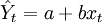$\hat{Y}_t=a+bx_t$　　　　（1）

式中，xt代表t期自变量的值；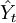$\hat{Y}_t$代表t期因变量的值；

a、b代表一元线性回归方程的参数。

a、b参数由下列公式求得（∑代表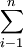$\sum^{n}_{i-1}$）：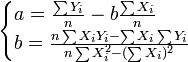$\begin{cases}a=\frac{\sum Y_i}{n}-b\frac{\sum X_i}{n}\\b=\frac{n\sum X_{i}Y_{i}-\sum X_i\sum Y_i}{n\sum X^2_i-(\sum X_i)^2} \end{cases}$

为简便计算，我们作以下定义：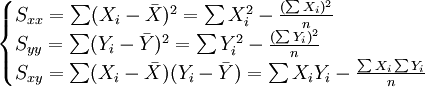$\begin{cases}S_{xx}=\sum(X_i-\bar{X})^2=\sum X^2_i-\frac{(\sum X_i)^2}{n}\\S_{yy}=\sum(Y_i-\bar{Y})^2=\sum Y^2_i-\frac{(\sum Y_i)^2}{n}\\S_{xy}=\sum(X_i-\bar{X})(Y_i-\bar{Y})=\sum X_i Y_i-\frac{\sum X_i\sum Y_i}{n}\end{cases}$　　　　(2)

式中：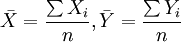$\bar{X}=\frac{\sum X_i}{n},\bar{Y}=\frac{\sum Y_i}{n}$

这样定义a、b后，参数由下列公式求得：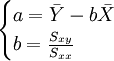$\begin{cases}a=\bar{Y}-b\bar{X}\\b=\frac{S_{xy}}{S_{xx}}\end{cases}$　　　　(3)

将a、b代入一元线性回归方程Yt = a + bxt，就可以建立预测模型，那么，只要给定xt值，即可求出预测值$\hat{Y}_t$。

在回归分析预测法中，需要对Ｘ、Ｙ之间相关程度作出判断，这就要计算相关系数r，其公式如下：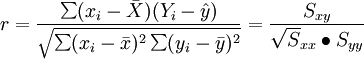$r=\frac{\sum(x_i-\bar{X})(Y_i-\hat{y})}{\sqrt{\sum(x_i-\bar{x})^2\sum(y_i-\bar{y})^2}}=\frac{S_{xy}}{\sqrt S_{xx}\bullet{S_{yy}}}$

相关系数r的特征有：

①相关系数取值范围为：-1≤r≤1 。

②r与b符合相同。当r>0，称正线性相关，Xi上升，Yi呈线性增加。当r<0，称负线性相关，Xi上升，Yi呈线性减少。

③|r|=0，X与Y无线性相关关系；|r|=1，完全确定的线性相关关系；0<|r|<1，X与Y存在一定的线性相关关系；|r|>0.7，为高度线性相关；0.3<|r|≤0.7，为中度线性相关；|r|≤0.3，为低度线性相关。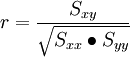$r=\frac{S_{xy}}{\sqrt{S_{xx}\bullet S_{yy}}}$　　　　(4)


展开全文• 2 回归分析 回归分析是确定两个或两个以上变量间相互依赖的定量关系的一种统计分析方法回归分析按照涉及的变量多少分为一元回归分析和多元回归分析按照自变量和因变量之间的关系类型可分为线性回归分析和非线性回归...
• “在现实中，多元回归分析是比一元回归分析更为常用方法，被大量用来解释各种经济现象，预测多种经济变量。” 解答： 注意：教材中p值和Excel数据分析包中的回归分析结果不一致，经排查，发现是教材...
发现一个规律：一本书，越到后面，实用性越强。
多元线性回归就是如此，感觉是个很实用的工具。
“在现实中，多元回归分析是比一元回归分析更为常用的方法，被大量用来解释各种经济现象，预测多种经济变量。”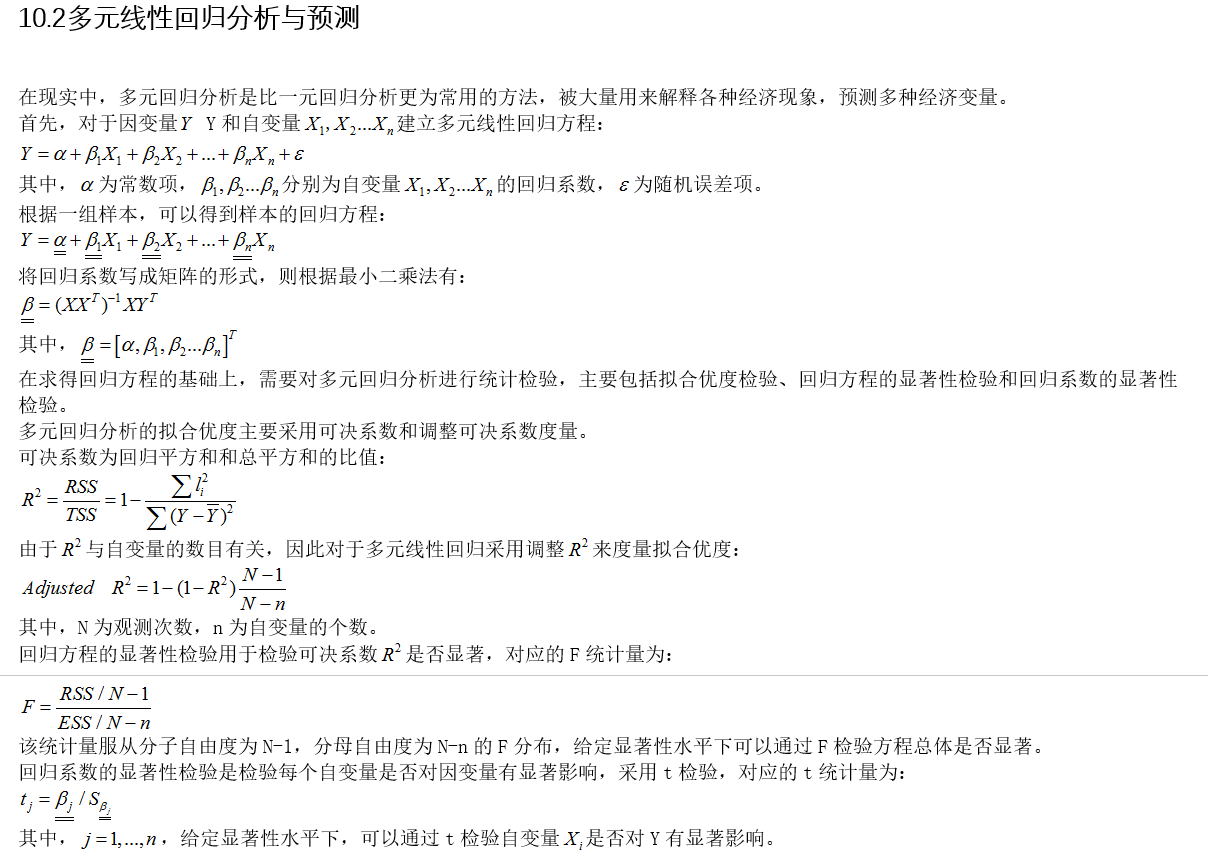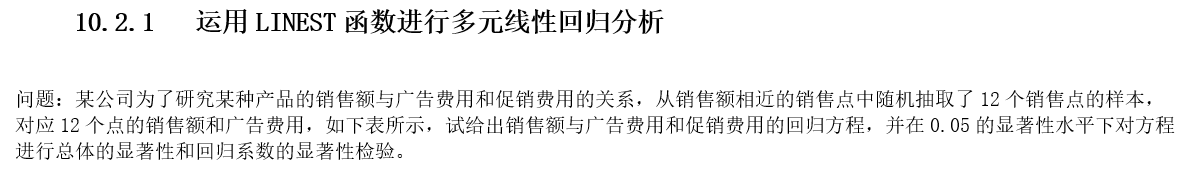解答：
注意：教材中p值和Excel数据分析包中的回归分析结果不一致，经排查，发现是教材中的自由度不对！在本文中已更正此错误。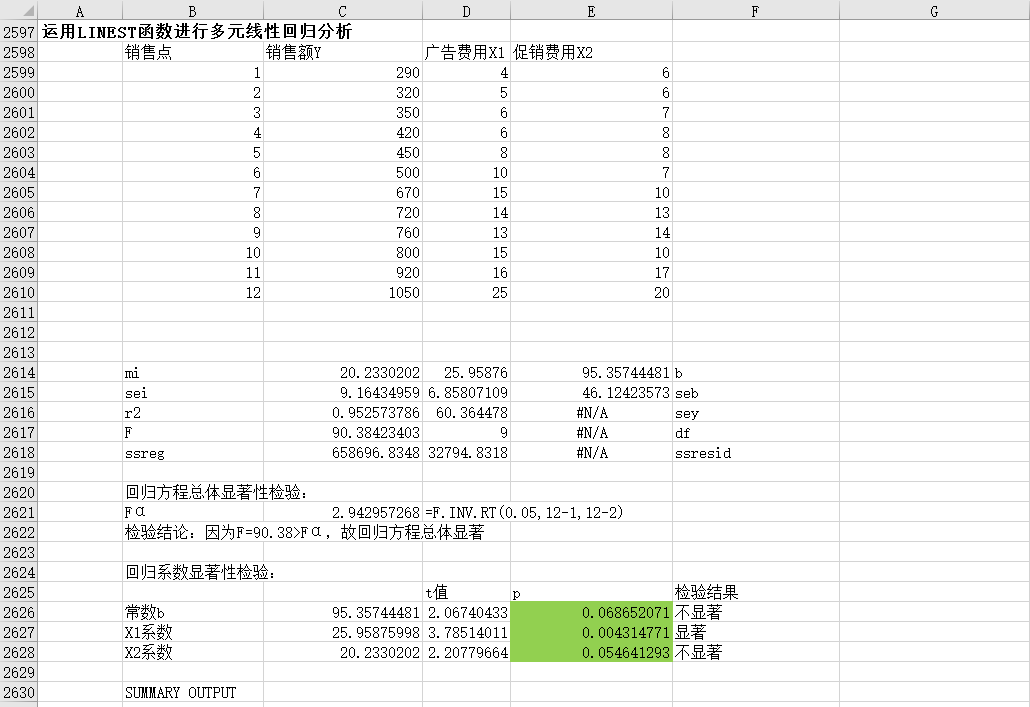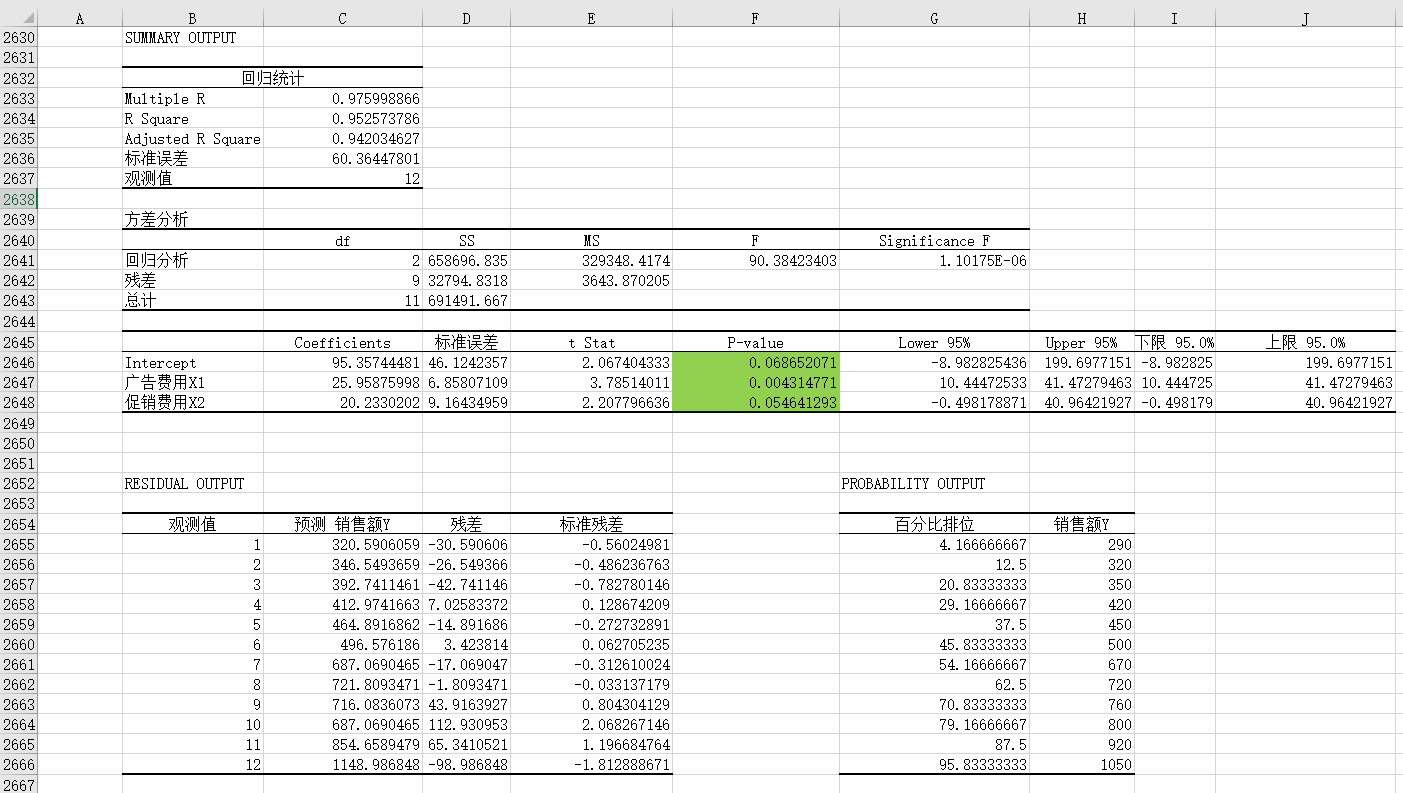﻿﻿

展开全文• 回归分析中，只包括一个自变量和一个因变量，且二者的关系可用一条直线近似表示，这种回归分析称为一元线性回归分析；如果回归分析中包括两个或两个以上的自变量，且因变量和自变量之间是线性关系，则称为多元线性...机器学习 梯度下降法 最小二乘法
• 理解 以a b为变量，预测值与真值的差的平方和为结果的函数 参数...包括他们的年收入 简单线性回归 简单线性回归的思想就是：假设你相信，这些男人的身高越高，年收入越高，然后找到一个一元线性方程 ，让这...
• 一元线性回归和多元线性回归的区别在于， 多元线性回归有（>1）个自变量， 而一元线性回归通常只有1个自变量。 最小二乘法也是用于拟合回归线最常用方法。 对于观测数据，它通过最小化每个数据点到线...算法
• ## Matlab 回归常用函数

千次阅读 多人点赞 2018-11-06 23:14:55
回归方法分类 一般回归方法 根据回归方法因变量的个数不同 根据回归函数的类型不同 一元、多元 ... Logistic 回归：是一种广义的线性回归分析模型，以指数结构函数作为回归模型的回归方法。  ...
• 如果在回归分析中，只包括一个自变量和一个因变量，且二者的关系可用一条直线近似表示，这种回归分析称为一元线性回归分析。 如果回归分析中包括两个或两个以上的自变量，且因变量和自变量之间是线性关系，则称为...机器学习 数据挖掘
• 按照自变量和因变量之间的关系类型，可分为线性回归分析和非线性回归分析。 线性回归（Linear Regression） 线性回归应该是最常用的回归了，其应用的原理就是最小二乘法。奔着少一点公式推导（不会推），多一点实例...r语言 数据可视化
• 以数据为基础而建立数学模型的方法称为数据建模方法， 包括回归...根据回归方法中因变量的个数和回归函数的类型（线性或非线性）可将回归方法分为:一元线性、一元非线性、多元回归。另外还有两种特殊的回归方式，一种在
• 文章目录统计平均值中位数众数线性回归一元多元回归逻辑回归正态分布决策树随机森林生存分析卡方检验 统计 平均值 语法 mean(x, trim = 0, na.rm = FALSE, ...) x - 是输入向量。 trim - 用于从排序的向量的两端...
• 主成分回归就是先对数据进行主成分分析，再进行多元线性回归。 主成分分析参考上篇博客，蹦篇博客介绍多元线性回归及其MATLAB实现。 多元线性回归是一种常用的...因此多元线性回归比一元线性回归的实用意义更大。...
• 根据回归方法中因变量个数和回归函数的类型（线性和非线性），可将回归方法分为一元线性回归、一元非线性回归和多元回归。 还有逐步回归即在回归过程中可以调整变量数量的回归方法；Logistic回归即以指数结构函数...数学建模
• 利用数理统计中的回归分析，来确定两个或以上变量之间相互依赖的变量关系的一种统计分析方法，只有一个自变量和一个因变量，两者之间的关系可以用线性表示，该回归分析一元线性回归，若包括两个及以上的自变量，自...算法 决策树 聚类 机器学习 人工智能
• 气象统计常用公式Chapter2 气象资料及其表示方法1. 方差2. 标准差3. 变率4. 变差系数5. 标准化变量6. 数据矩阵7. 均值向量8.... 一元线性回归2. 多元线性回归Chapter5 气候趋势分析1. 滑动平均2. 累积距平3.
• 以数据为基础而建立数学模型的方法称为数据...根据回归方法中因变量的个数和回归函数的类型（线性或非线性）可将回归方法分为:一元线性、一元非线性、多元回归。另外还有两种特殊的回归方式，一种在回归过程中可以...
• MATLAB数学建模方法与实践（第3版）——读书笔记1.数学建模的五类问题2....相关系数降维3.2常用的数据建模方法3.2.1回归一元线性回归一元非线性回归多元回归逐步回归logistic回归(预测)3.2.2机器学习分类聚类小结3.2数学建模 matlab
• ## C++常用算法程序集

热门讨论 2011-03-24 09:15:32
8.2 一元线性回归分析499 8.3 多元线性回归分析503 8.4 逐步回归分析510 8.5 半对数数据相关521 8.6 对数数据相关525第9章 极值问题的求解529 9.1 一维极值连分式法529 9.2 n维极值连分式法532 9.3 不等式约束...
• Day2_Simple_Linear_Regression(一元线性回归) 本文引用自 Simple Linear Regression, 对其中内容进行了评注与补充说明。 回归分析是一种预测性的建模技术，它研究的是因变量（目标）和自变量（预测器）之间的...
• 11.2 一元线性回归分析 11.3 多元线性回归分析 11.4 逐步回归分析 11.5 半对数数据相关 11.6 对数数据相关 第12章 极值问题的求解 12.1 一维极值连分式法 12.1 n维维极值连分式法 12.3 不等式约束线性规划问 12.4 ...
• 接下来的几篇文章会给大家介绍一些线性模型，一元线性回归，多元线性回归，广义线性模型中的逻辑回归，由Fisher判别分析演变而来的线性判别分析，其中的线性判别分析会用到拉格朗日乘子法和矩阵奇异值，矩阵特征值...
• 　本书主要内容是：多元正态分布、方差分析、回归分析、因子分析与线性模型、聚类分析和统计量的分布．附录中列出了常用的多元分布表． 　读者对象是高等学校数学系教师、高年级学生，应用多元统计的科技工作者．
• 线性回归分析模型 1.线性回归模型的原理、分析步骤、操作和结果阅读 2.逐步、前进、后退三种筛选方法的含义与操作用法、分析结果的解读 3.各种常用模型诊断工具（分类图等）的操作使用 4.加权最小二乘法、两阶段...
• 8.2 一元线性回归分析499 8.3 多元线性回归分析503 8.4 逐步回归分析510 8.5 半对数数据相关521 8.6 对数数据相关525第9章 极值问题的求解529 9.1 一维极值连分式法529 9.2 n维极值连分式法532 9.3 不等式约束...
• 11.2 一元线性回归分析 11.3 多元线性回归分析 11.4 逐步回归分析 11.5 半对数数据相关 11.6 对数数据相关 第12章 极值问题的求解 12.1 一维极值连分式法 12.1 n维维极值连分式法 12.3 不等式约束线性规划问 12.4 ...
• 10.11 全区间积分的哈明方法 10.12 积分一步的特雷纳方法 10.13 积分刚性方程组的吉尔方法 10.14 二阶微分方程边值问题的数值解法 第11章 数据处理 11.1 随机样本分析 11.2 一元线性回归分析 11.3 多元线性回归分析 ...
• 11.2 一元线性回归分析 11.3 多元线性回归分析 11.4 逐步回归分析 11.5 半对数数据相关 11.6 对数数据相关 第12章 极值问题的求解 12.1 一维极值连分式法 12.1 n维维极值连分式法 12.3 不等式约束线性规划问 12.4 ...
• 11.2 一元线性回归分析 11.3 多元线性回归分析 11.4 逐步回归分析 11.5 半对数数据相关 11.6 对数数据相关 第12章 极值问题的求解 12.1 一维极值连分式法 12.1 n维维极值连分式法 12.3 不等式约束线性规划问 12.4 ...